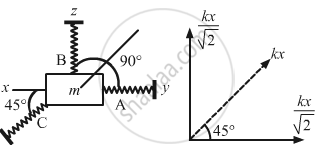Advertisement Remove all ads

# A Particle of Mass M is Attatched to Three Springs A, B and C of Equal Force Constants Kas Shown in Figure . - Physics

Sum

A particle of mass m is attatched to three springs A, B and C of equal force constants kas shown in figure . If the particle is pushed slightly against the spring C and released, find the time period of oscillation.Advertisement Remove all ads

#### Solution

(a) Let us push the particle lightly against the spring C through displacement x.As a result of this movement, the resultant force on the particle is kx​.
The force on the particle due to springs A and B is $\frac{kx}{\sqrt{2}}$ .

Total Resultant force $= kx + \sqrt{\left( \frac{kx}{\sqrt{2}} \right)^2 + \left( \frac{kx}{\sqrt{2}} \right)^2}$

kx + kx = 2kx

Acceleration is given by $= \frac{2kx}{m}$

$\text { Time period }= 2\pi\sqrt{\frac{\text { Displacement }}{\text { Acceleration }}}$

$= 2\pi\sqrt{\frac{x}{2kx/m}}$

$= 2\pi\sqrt{\frac{m}{2k}}$

Is there an error in this question or solution?
Advertisement Remove all ads

#### APPEARS IN

HC Verma Class 11, 12 Concepts of Physics 1
Chapter 12 Simple Harmonics Motion
Q 19 | Page 253
Advertisement Remove all ads

#### Video TutorialsVIEW ALL 

Advertisement Remove all ads
Share
Notifications

View all notifications

Forgot password?